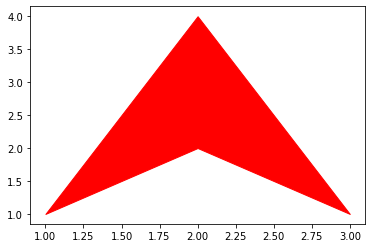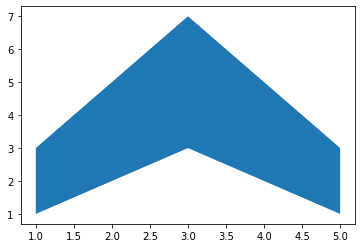Open In App

# Make filled polygons between two horizontal curves in Python using Matplotlib

Matplotlib is an amazing visualization library in Python for 2D plots of arrays. Matplotlib is a multi-platform data visualization library built on NumPy arrays and designed to work with the broader SciPy stack.

To create filled polygons between two curves a PolyCollection filling needs to be created between y1 and y2.

• x: It is an array of length N that holds data of x.
• y1: It is an array or a scalar of length N that holds data of y.
• y2: It is an array or a scalar of length N that holds data of y.

Below is the implementation.

 `import` `matplotlib.pyplot as plt``  ` `     ` `x_inp``=` `input``(``"Enter values of X array:  "``).split()``x``=` `[``int``(i) ``for` `i ``in` `x_inp]`` ` `y1_inp``=` `input``(``"Enter values of Y1 array:  "``).split()``y1``=` `[``int``(i) ``for` `i ``in` `y1_inp]`` ` `y2_inp``=` `input``(``"Enter values of Y2 array:  "``).split()``y2``=` `[``int``(i) ``for` `i ``in` `y2_inp]``  ` `color``=``input``(``"Enter color:  "``)`` ` `plt.fill_between(x, y1, y2, color ``=` `color)``plt.show()`

For input:

```Enter values of X array:  1 2 3
Enter values of Y1 array:  1 2 1
Enter values of Y2 array:  1 4 1
Enter color:  red
```

Output :For input:

```Enter values of X array:  1 2 3 4 5
Enter values of Y1 array:  1 2 3 2 1
Enter values of Y2 array:  3 5 7 5 3
Enter color:  blue
```

Output :